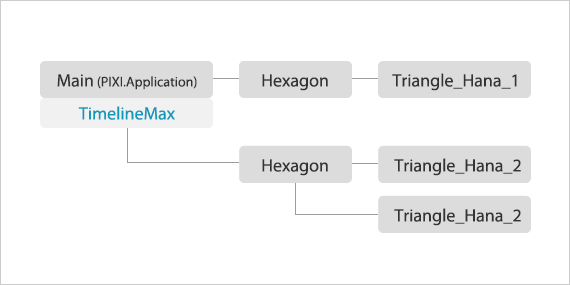#2018 iT 邦幫忙鐵人賽DAY 29
2
Modern Web

## [PixiJS - Day-29] 實作：第二版萬花筒

- 架構：

- 萬花筒內花瓣動態控制：

- 畫面更新與場景控制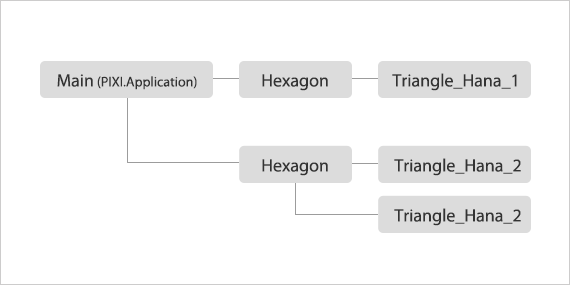• 主程式：`Main`，控制 `PIXI.Application` 實體的程式
• 六邊形：`Hexagon`
• 花：`Triangle_Hana_1~4` 等各種不同花的實作

- 主程式

• 決定目前場景上會有幾個六邊形，以及六邊形裡是哪一種花
• 控制目前播放的片段

- 六邊形

• Triangle_Hana_1
• Triangle_Hana_2
• Triangle_Hana_3
...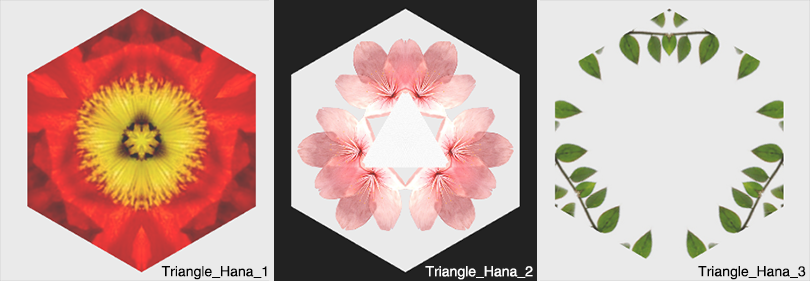``````// main.js
var hexagon = new Hexagon("hana_1");

// hexagon.js
Hexagon = function(\$type){
switch(\$type){
case "hana_1":
this.triangle1 = new Triangle_Hana_1();
this.triangle2 = new Triangle_Hana_1();
this.triangle3 = new Triangle_Hana_1();
this.triangle4 = new Triangle_Hana_1();
this.triangle5 = new Triangle_Hana_1();
this.triangle6 = new Triangle_Hana_1();
break;
...
}
}

// hana_1.js
this.texture = PIXI.Texture.fromImage('assets/hana/hana_1_2.png');
...

``````

- 花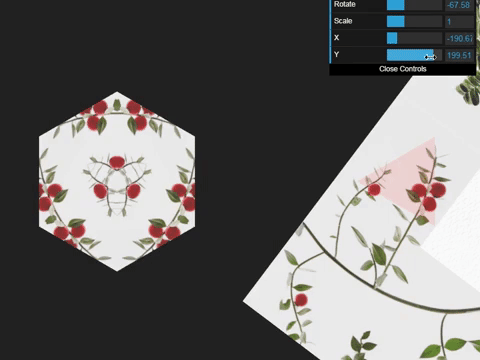• 左邊：萬花筒六邊形完整樣貌
• 右邊：是六邊形的其中一個三角形，半透明紅色三角形是遮罩範圍但不設定遮罩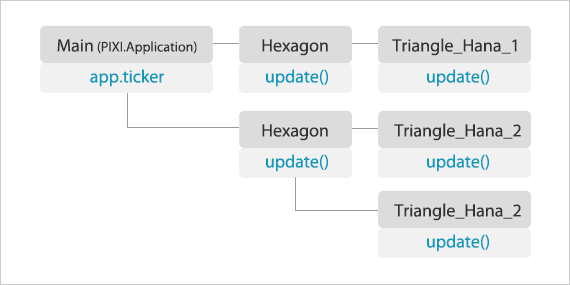app.ticker 會隨著時間一直呼叫